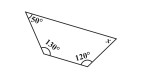"
">

# Find x."

Given :

Three angles of a quadrilateral are 50°, 130°, 120°.

To do :

We have to find the measure of angle x.

Solution :

Sum of the angles in a quadrilateral is 360 degrees.

Therefore,

$50°+130°+120°+x=360°$

$x+300°=360°$

$x=360°-300°$

$x=60°$

Therefore, the measure of x is 60°.

Updated on: 10-Oct-2022

10 Views